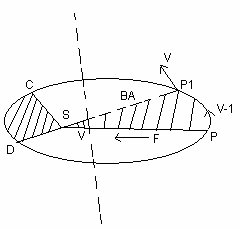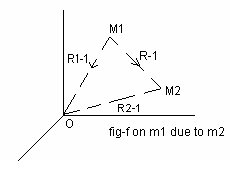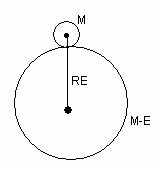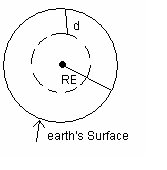# Gravitation

## (1) Introduction :

• In our dairy life we have noticed things falling freely downwords towards earth when thrown upwards or dispped from some hight.
• Fact that all bodies irrespective of their masses are accelerated towards the earth mith a constant aceeleation was first recognized by galive (1564-1642)
• The motion of celesh'al bodies such as moon. earth plametes etc. and attrachieve of moon towards earth and arth towards sun is an interasting subject of study since long time.
• Now the question's what is the force that produces such acceleration is which earth attract all bodies      towards the centre and what is the law governing this force.
• Is this law is same for both earthly and weshal bodies.
• Answer to this question was given by Newton as he declared that "laws of nature are same for earthly and weshal Bodies".
• The force between any object falling freely towards earth and that between earth and moon are gowerned by the same laws.
• Johnaase kepler (1571-1631) Studied the planetary motion in detail and formulated his three laws of      planetary motion, which were available Universal law of grawitation.

## (2) Kepler's Law :-

Kepler's law of planetary motion are :-

### (i)Law of orbits :-

Each planet revolues around the sun in an elliptical orbit with sun at one of the foci of the ellipse asd     shown in fig (a) below.

Fig (a) An ellipse traced by planet sevolary round the sun.AO = a - Sewi major axis
BO = b - Sewi minor axis
P - hearest point between planet and sun k/as perihetion
A - farthest point between planet and sun apheiton.

### (ii)Law of areas :-

The line joining planet and the sun sweeps equal area in equal intervals of time" [fig b]

This law follows from the observation that when planet is nearer to the sun its velocity increases and It appears to be slower when it is farther from the sun.

### (iii)Law of periods :-

The squre of time period of any planet about the sun is propotional to the cube of the semi-major axis."
• If T is the time period of semi major axis a of elliptical orbit then.
T2 x a3                         (1)
• If T1 and T2 are time periods of any two planets and a1 and a2 being their semi major axis resp. then
T12 x a13 = a13
T22 x a23 a23                (2)
This question (2) can be used to find the time period of a planet, when the time period of the other
planet and the semi-major axis of orbits of two planets.

## (3) Universal law of gravition :-

• Everybody in the universe attracts every other body with a force which is directly proportional to the
product of their masses and invessly proportional to the square of distance between them.
• Mathematically Newton's gravitation law is if F is the force acting between two bodies of masses M1 and M2 and the distance between them is R then majnitade of force is given as$$F=\frac{Gm_{1}m_{2}}{r^{2}}$$
In vector notation
$$F=\frac{Gm_{1}m_{2}(-\hat{\boldsymbol{\mathbf{r}}})}{r^{2}}$$
$$F=-\frac{Gm_{1}m_{2}\hat{\boldsymbol{\mathbf{r}}}}{r^{2}}$$      where G- universal gravitational constant
$$\hat{\boldsymbol{\mathbf{r}}}$$ - unit vector from m1 to m2 and $$\hat{\boldsymbol{\mathbf{r}}} = \hat{\boldsymbol{\mathbf{r_{1}}}}^ - \hat{\boldsymbol{\mathbf{r_{2}}}}$$
Gravitational force constant is
SI -           G= 6.67 x 10-11 nm2 kg-2
CGS -           G= 6.67 x 10-8 dyn cm2 g-2
Deminsional formula of C1 is [m-1L3T-2]

## (4) Acceleration due to gravity of earth :-

• Earth attracts every object lying an its surface towards its centre with a force known as gravitational towards its centre with a force known as gravitational pull or gravity.
• Whenever force acts on any body it produces acceleration and in case of gravitation this acceleration produced under effect of gravity is known as accelesation due to gravity (g)
• Value of accelesation due to gravity is independent of mass of the body and its value near surface of earth is 9.8 ms-2
• Expression for acceleration due to gravity
Consider mass of earth to be as ME and its redius be RE Suppose a body of mass M (much smaller then that fo earth) is kept at the earth surface. Force eseerted by earth on the body of mass m is
$$F=\frac{-GMM_{E}}{R_{E}^{2}}$$
The force for the body due to earth produces acceleration due to      gravity
(g) in the motion of the body. From Newton's Second law of motion
f=mg
from (4) and (s)
$$g=\frac{-GM_{E}}{R_{E}^{2}}$$
which is acceleration due to gravity at earth's surface.

## (5) Acceleration due to gravity below and above the earth surface :-

(i)     Above earth's surface• An object of mass m is placed at hight h above the earth's active in this object is
$$F=\frac{-GMM_{E}}{(R_{E}+h)^{2}}$$
From this it can be concluded that value of g decreases as distance above surface of earth increases now,
$$g=\frac{-GM_{E}}{R_{E}(1+\frac{h}{R_{E}})^{2}}$$
g=\frac{g_{0}}{(1+\frac{h}{R_{E}})^{2}}
$$g_{0}=\frac{-GM_{E}}{R_{E}^{2}}$$
• eqn (7) tells us that for small hight h above surface of earth. value of g decreases by factor (1-2h/RE)
-     for h<<R
g=go(1+h/RE)-2
g=go(1-2h/RE) Expanding by Binomial theriom

(ii)     Below the earth's surface• If one goes inside the earth surface the value of g again decreases
• P = density of material of earth them
m=(4/3)(RE)3P
From this acceleration due to gravity at earth�s Surface is
$$g_{0}=\frac{G(4/3)R_{E}^{3}P}{R_{E}^{2}}$$
g=(4/3)GREP ---------(8)
g-acceleration due to gravity at depth D below earth's surface
-Body at depth d will experience force only due to portion of reduce (RE-d) of earth's
-outer spherical shell of thickness d will not exeperience any force
-M is mas of the portion of earth with radius (RE-d) then
$$g=\frac{-GM}{r^{2}}$$
M=(4/3)(RE-d)3 P
$$g=\frac{G(4/3)(R_{E}-d)^{3}P}{(R_{E}-d)^{2}}$$
g= (4/3)G (RE-d)P                    (9)
Dividing epn (9) by (8)
g/go= (1-d/RE)
or g=go (1-d/RE)
nbsp;                       (10)
from epn (10) is cleaer that acceeleration due to gravity also decreases with depth.